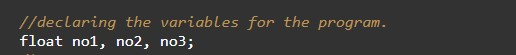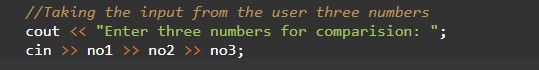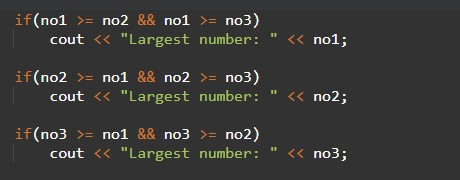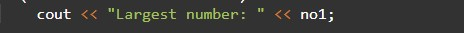# C++ Program to Find Largest Number Among Three Numbers

In this tutorial you will learn about the C++ Program to Find Largest Number Among Three Numbers and its application with practical example.

## C++ Program to Find Largest Number Among Three Numbers

In this tutorial, we will learn to create a C++ program that will find Largest Number Among Three Numbers using C++ programming.

## Prerequisites

Before starting with this tutorial we assume that you are best aware of the following C++ programming topics:

• Operators in C++ Programming.
• Basic Input and Output function in C++ Programming.
• Basic C++ programming.
• Conditional statement in C++ programming.

## Program to Find Largest Number Among Three Numbers

In c++ programming, it is possible to take numerical input from the user and find Largest Number Among Three Numbers with the help of a very small amount of code. The C++ language has many types of header libraries which has supported function in them with the help of these files the programming is easy. But today we will find Largest Number Among Three Numbers using c++ programming.

Algorithm:-

With the help of this program, we can find Largest Number Among Three Numbers.

## Output:-In the above program, we have first initialized the required variable.• no1 = it will hold the integer value.
• no2 = it will hold the integer value.
• no3 = it will hold the integer value.

Input numbers for the program.Program Logic Code.Printing output for the greatest number.In this tutorial we have learn about the C++ Program to Find Largest Number Among Three Numbers and its application with practical example. I hope you will like this tutorial.